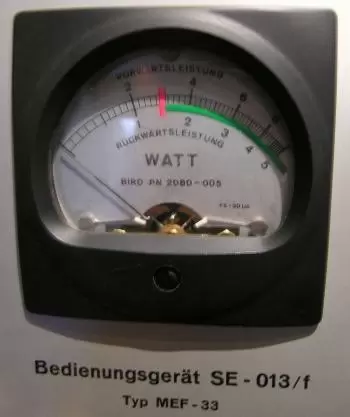# What Is a Watt?

The watt unit is the power unit according to the international system of units; the watt symbol is the letter W. Power is the rate at which energy is expended.It is the unit used to quantify the amount of energy transferred in one second: one watt is equal to one joule (J) per second (s).

1W = 1J/s

The watt is usually associated with the unit of electrical power, that is, linked to electrical energy. However, power units can also refer to any power: mechanical, acoustic, or magnetic.

In mechanics, the watt is the power developed by a force of one newton applied to the point that moves one meter during one second. It means that if the point on which a force of one newton is applied is moving at a speed of 1 m / s, the power is equal to 1 watt:

1W = 1N·1m / 1s

It can also be expressed as the amount of work done in one second.

In electricity, a watt is used to measure the power produced by a difference in electric potential in volts (V) and the intensity of electric current (A) that passes through a conductor, that is:

W = VA

James Watt (1736-1819), the steam engine's inventor, accepted it as the unit of the international SI system of units.

Other units related to power are horsepower and horsepower.

The term watt comes from watt, named after James Watt, and was adopted by the Second Congress of the British Association for the Advancement of Science in 1889. In 1960, the Eleventh General Conference on Weights and Measures incorporated it into the System Units International.

## What Is the Difference Between Watts and Watt-hours?

The power is measured in watts, and watt-hours (Wh) are units to measure the energy that, in this case, do not belong to the international system of units (SI).

The kilowatt-hour (kWh) equal to 1,000 watt-hours and 3600 kilojoules. The kilowatt-hour is commonly used as a billing unit for energy delivered to consumers by electric utilities. In the same way, a megawatt-hour (Mwh) is equal to 1,000 Kilowatt-hours (Kwh).

A kilowatt-hour is 1000 watts used for one hour. For example, the power consumption of a 100-watt light bulb operating for ten hours is one kilowatt-hour.

## What Are Megawatts (MW)?

One megawatt equals one million watts.

Megawatts are used when very high power units have to be expressed. In the nuclear power sector, it is common to speak of megawatts referring to a nuclear plant's power.

Many applications can be found where the transfer, generation or consumption of energy is on this scale. Some of the possibilities can even be found in nature, such as electrical discharges from lightning.

Examples of machinery that works with powers of this order are, for example, large electric motors, large ships, special machinery.

A large residential or commercial building can consume a few megawatts of electrical power and energy for heating.

## Electric Megawatt

The electric megawatt (represented by MWe) is a term used in electrical power generation to refer to electrical power.

It is common for a thermal power plant to talk about thermal power and electrical power. In this case, the thermal power corresponds to the amount of thermal energy that is generated per second (MWt)

Subsequently, the power plant transforms this energy into electricity. The power output is expressed in electrical megawatts. The MWe value is always lower than the MWt due to the energy losses of the process.

## Power Examples

If a person removes a 100-kilogram mass from a 3-meter ladder in 5 seconds, his work is approximately 3,000 (j = Nm).

A mid-volume car engine is 50-100 kilowatts (kW). Larger or higher-performing vehicles have more powerful engines.

An average nuclear power plant produces 800-900 MW (megawatts).

Author:

Published: October 29, 2018
Last review: December 3, 2020